×

Search anything:

# Linked List with no NULLs

#### Algorithms Data Structures Software Engineering linked listA Linked list is a dynamic data structure which can grow or shrink on demand. It is usually implemented using NULLs, we will consider an alternative no NULL approach and use placeholder nodes.

# Introduction

A linked list is a dynamic data structure made up of a sequence of nodes. These nodes are not stored contiguously in memory but instead each nodes stores a link to the next node. Thus each node has a link that points to the next node in the list.
In the implementation, the last node usually points to a `NULL` value. But we will use a placeholder node. The number of nodes in a linked list is not fixed and thus the list can grow or shrink on demand. This dynamic nature is very useful when we need to deal with an unknown number of objects.
A linked list is simply a sequence of nodes. Among all the nodes in the linked list the first node is specified as a `head` node, and the last node will be the `tail` node. Note that in-case the list only has one node, the head and tail node will be the same node. To specify these we use `head` and `tail` node pointers.

# Node design

A linked list is made up of nodes of the same type. Here we'll assume that we want to store integer values in the linked list. We will consider the nodes to be of two types: list nodes and placeholder nodes.
The list nodes each have a data variable called `value` and a `next` pointer.
The placeholder nodes are used like the `NULL` values and are used to indicate the end of the list.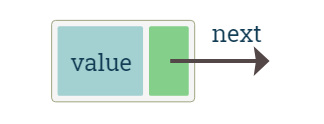Diagram shows the list node

The list node is implemented as a `struct` which has an integer value variable alongwith the pointer which will store the address of the next node. For convenience a constructor has also been provided. Note that this is valid in C++.

``````struct node
{
int value;
node* next;
node(int value): value(value), next(nullptr)
{
}
};
``````

A node would be created by dynamic memory allocation. Free memory is allocated using the `new` operator. We'll use the following helper function to create a `node`.

``````node* createNode(int value)
{
return new node(value);
}
``````

# Placeholder node

The last element's `next` pointer points to the placeholder node. This is analogous to the using a `NULL` value. The palceholder node has the value of `INT_MAX`. Since we'll be using nodes themselves to indicate the end of the list, we need a way to differentiate them. We could assume that their values are positive infinity (or negative infinity). In this implementation we use use the value of macro `INT_MAX` defined in the header `<climits>`.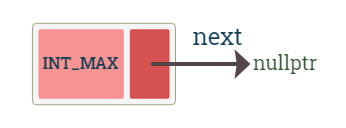diagram shows a placeholder node

We use a helper function to create the node. The function returns the address of the newly allocated placeholder node.

``````static node* getPlaceholderNode()
{
return new node(INT_MAX);
}
``````

# Size of the linked list

The size of the linked list is the number of nodes in the list. To determine the size, we use a `size` variable. Initially at the time of creation of the linked list the value of this variable is set to `0`. The value is incremented when a new node is added to the linked list and decremented when an existing node is deleted from it.
The use of this variable allows us to get the size in `O(1)` (constant) time by simply returning the value of the variable `size`. We'll use a function `getSize()` to return the size.

``````int getSize()
{
return this->size;
}
``````

The linked list is empty when there are no nodes in the list. As for our implementation, the empty list will have only a placeholder node and the size of the linked list would be 0. Additionally the `head` and the `tail` pointers would point to this placeholder node.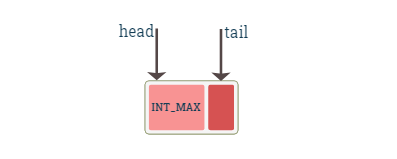The diagram shows an empty linked list

# Inserting a node

We consider three ways to insert a node:

1. At the beginning of the list
2. At the end of the list
3. At any index in the list

## Inserting at the beginning of the list

Inserting at the beginning is easy. We allocate a new node and then make its `next` pointer point to the first node of the existing linked list. Next we make the newly inserted node the new head node of the list.
To insert we can have two cases:

1. The linked list is empty
2. The linked list has one or more nodes

Insertion at the beginning when the list is empty
The linked list is empty which means that the list has only a placeholder node. The `head` and `tail` pointers point to this node. To insert the node we first create the node, make its `next` pointer point to the placeholder nodes. Then finally make the `head` and `tail` node to point to this newly inserted node. Thus after insertion, this newly created node will be both the head node and the tail node.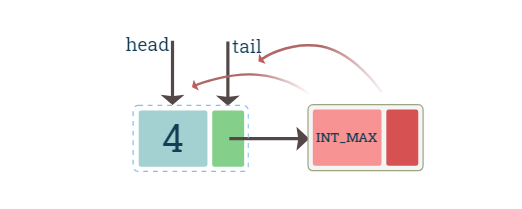Insertion at the beginning when the list is non-empty
The linked list is not empty which means that there is atleast one node. we need to create the node and make its `next` pointer point the head node. Then make the `head` pointer point to this newly inserted node. Here we need not change the `tail` pointer.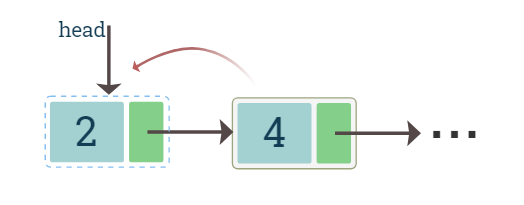Notice that in both the cases, the newly inserted node is made the head node and `tail` pointer is changed only for the second case. So both cases can be combined and implemented as follows

``````void add_first(int value)
{
// create the new node
node* n = new node(value);

// if list is empty, we have to change tail to point to this new node
{
tail = n;
}

// in both the cases, the head node will be the newly inserted node

// update size
size++;
}
``````

## Inserting at the end of the list

Similar to adding node at front, adding at the end also has two cases:

1. Either the linked list is empty
2. It has one or more nodes

Insertion at the end when the list is empty
This is equivalent to inserting at the beginning when the list is empty.

Insertion at the end when the list is non-empty
For a non-empty list we need a pointer to both the last node and the placeholder node. For insertion the last node's `next` pointer would point to the new node and the new node's `next` pointer will point to the placeholder node.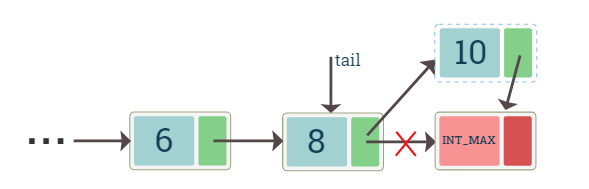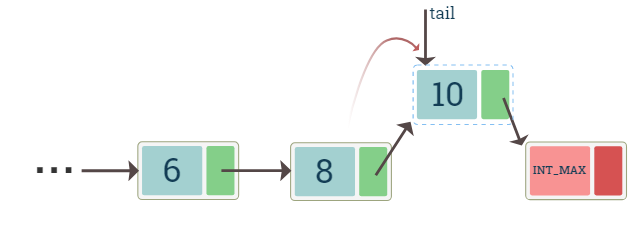diagrams show insertion of a node at the end of the list

Both of the above cases can be combined and implemented as follows

``````void add_last(int value)
{
// create the new node
node* n = new node(value);

// if the list is empty
{
n->next = tail;
tail = n;
}
else // else list is not empty
{
// pn is pointer to the placeholder node
node* pn = tail->next;

// insert after tail and before placeholder node
tail->next = n;
n->next = pn;

// make this node the new tail node
tail = n;
}
// update size
size++;
}
``````

# Deleting a node

Deleting a node is not simple as every node will in addition to value, store the pointer to the next node. If we delete some node directly then the memory address of the next node is lost.

What if the node doesn't exist?
There are multiple ways to handle this. For simplicity, in our implementation we do nothing if a node or a position in the linked list doesn't exist. So if we try to delete a node at position 0 in an empty list, there won't be any error messages. This can be easily changed however.

We consider three cases for the deletion of a node.

1. at the beginning of the linked list
2. at the end of the linked list
3. at any position in the list

## Deletion at the beginning

The node that we want to delete is the one that is pointed to by the `head` pointer. We assign a `current` pointer to this node and then move the `head` pointer to the next node. Now the `current` pointer is pointing to the node that we want to delete and the `head` pointer is pointing to the new head node. We can now simply delete the `current` pointer node.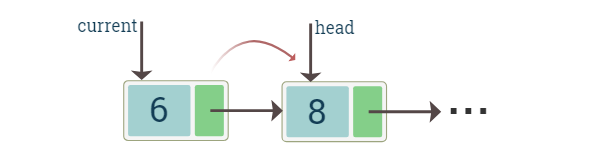diagram shows deletion of the head node

``````void remove_first()
{
if(getSize()>0)
{
delete current;
if(getSize()==1)
{
}
size--;
}
}
``````

## Deletion at the end

To delete the node at the end we first make the `current` pointer point to it. we also need a pointer to the previous node. To delete we set the `next` of the previous node to the placeholder node, and then we can simply delete the current node.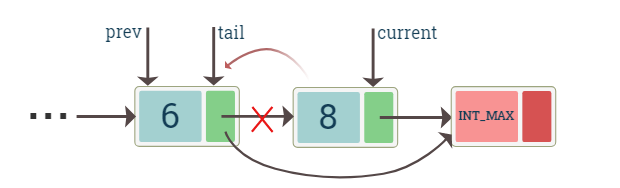The diagram shows the deletion of the tail node

``````void remove_last()
{
if(getSize()>0)
{
if(getSize()==1)
{
tail = current->next;
delete current;
size--;
}
else
{
for(int i=0; i<getSize()-2; ++i)
{
prev = prev->next;
}
node* current = prev->next;
prev->next = current->next;
tail = prev;
delete current;
size--;
}
}
}
``````

## Deletion at any position

If we want to delete a head node (position `0`) or the tail node (position `size-1`) then we can simply use the functions `remove_first()` and `remove_last()` respectively.
When we want to delete a node in other positions, we can assume that a previous and next node (to the current node) always exists. We point the `prev` to the previous node and `current` to the node that we want to delete. Then next node can be accessed using `current->next`.
After this we can set the `prev`'s `next` pointer to the next node, and then simply delete the current node.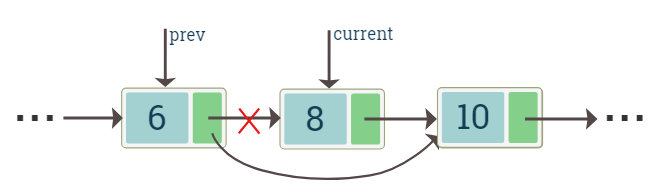The diagram shows deletion of a node

``````void remove_at(int idx)
{
if(idx >= 0 && idx < getSize())
{
if(idx == 0)
{
remove_first();
}
else if(idx == getSize()-1)
{
remove_last();
}
else{
for(int i = 0; i<idx-1; ++i)
prev = prev->next;
node* current = prev->next;
prev->next = current->next;
delete current;
size--;
}
}
}
``````

# Conclusion

While the important functions were covered for the placeholder nodes based Linked list. We can add a constructor and destructor and other functions. The complete implementation is at https://github.com/RohitTopi/opengenus/blob/main/Linked_list_no_NULL.cpp

#### Rohit Topi

Intern at OpenGenus | B.Tech Computer Science Student at KLS Gogte Institute Of Technology Belgaum | Contributor to OpenGenus book: "Binary Tree Problems: Must for Interviews and Competitive Coding"